# XLOOKUP

The XLOOKUP function returns the value in the same row after finding a matching value in any column.
This looks up a value in any column of a table and returns a value in the same row but in a different column.
Alternatively it can look up a value in one row and return a value in the same column but in a different row.
This function is not case sensitive.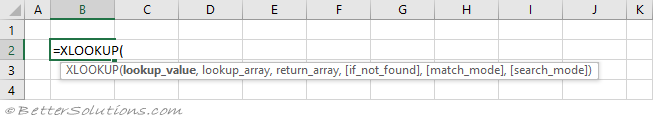### Replaces VLOOKUP and HLOOKUP

This function replaces both VLOOKUP and HLOOKUP.
This function defaults to "exact match" and not "closest match or the next smallest".
This function still works after inserting or deleting columns (or rows).
This function can lookup values on the left of the lookup_value column.
This function can search from the bottom up without sorting.

### Return the Exact Match (vertical, unique values)

This can be used instead of the VLOOKUP function
When the "match_mode" argument is 0 an exact match is found.
If there is more than one occurrence, the first occurrence is found.
The definition of the first occurrence is the same regardless of whether the table is sorted.
The table does not have to be sorted when you are looking for an exact match.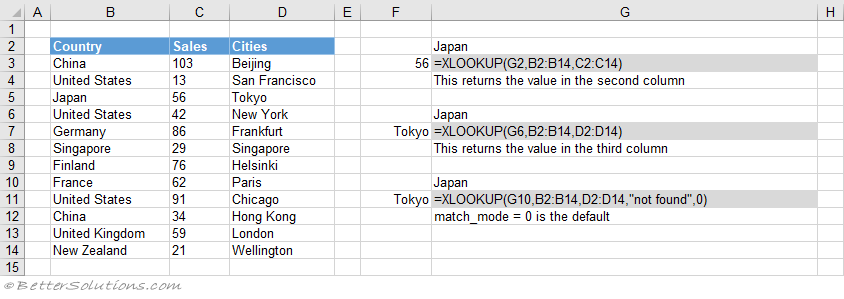### Return the Exact Match (horizontal, unique values)

This can be used instead of the HLOOKUP function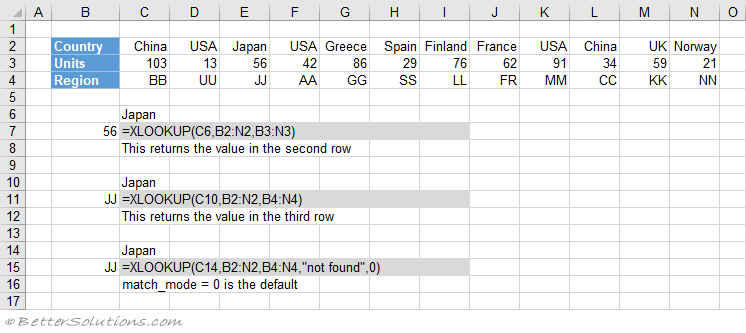### Return the Exact Match (item does not exist)

When the "lookup_value" is not in the list #N/A is returned.
When the "match_mode" argument is 0 an exact match is found. This is the default.
The table does not have to be sorted when looking for an exact match.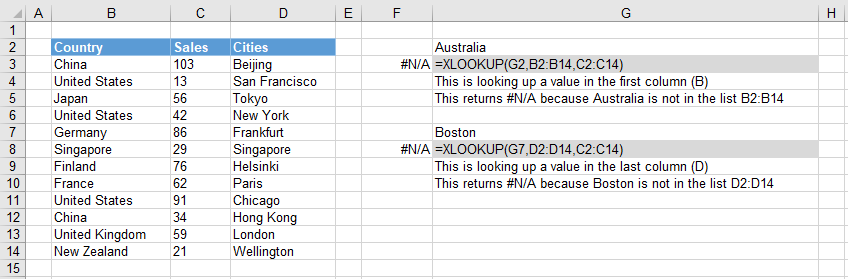### Return the Exact Match (default value)

When the "if_not_found" argument is provided this value will be returned when no match is found.
When the "match_mode" argument is 0 an exact match is found. This is the default.
The table does not have to be sorted when looking for an exact match.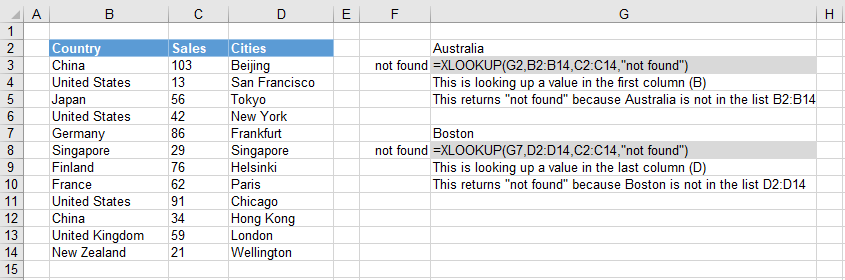### Return the Exact Match (case sensitive, EXACT)

The XLOOKUP function is not case sensitive.
This means that upper case and lower case characters are equal (for example Japan = JAPAN).
You can perform a case sensitive lookup by using the EXACT function.
The table does not have to be sorted when looking for an exact match.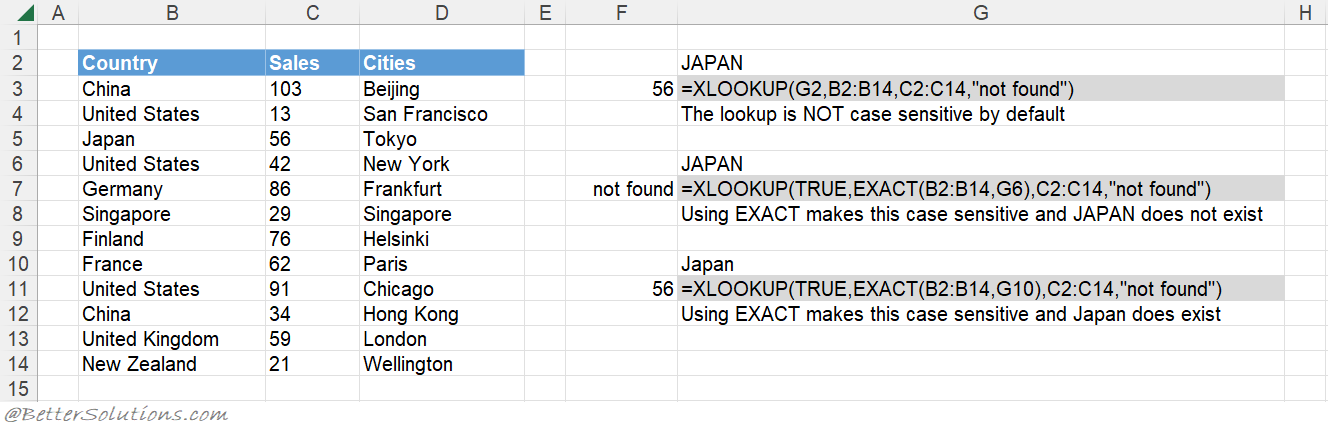### Return the Exact Match (first occurrence, sorted or not sorted)

This function will return the first occurrence starting at the top.
When the "match_mode" argument is 0 an exact match is found. This is the default.
When the "search_mode" argument is 1 the first occurrence is found. This is the default.
The table does not have to be sorted to return the first occurrence.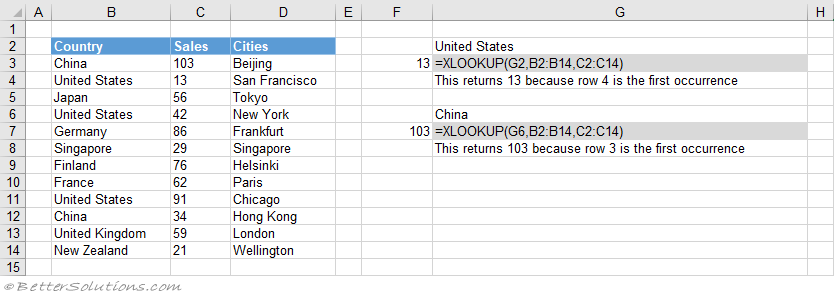### Return the Exact Match (last occurrence, sorted)

The last occurrence can be achieved when the "lookup_value" column is sorted into Ascending Order.
When the "match_mode" argument is 0 an exact match is found. This is the default.
When the "search_mode" argument is -1 the last occurrence is found.
The table has been sorted by Country and then by Sales.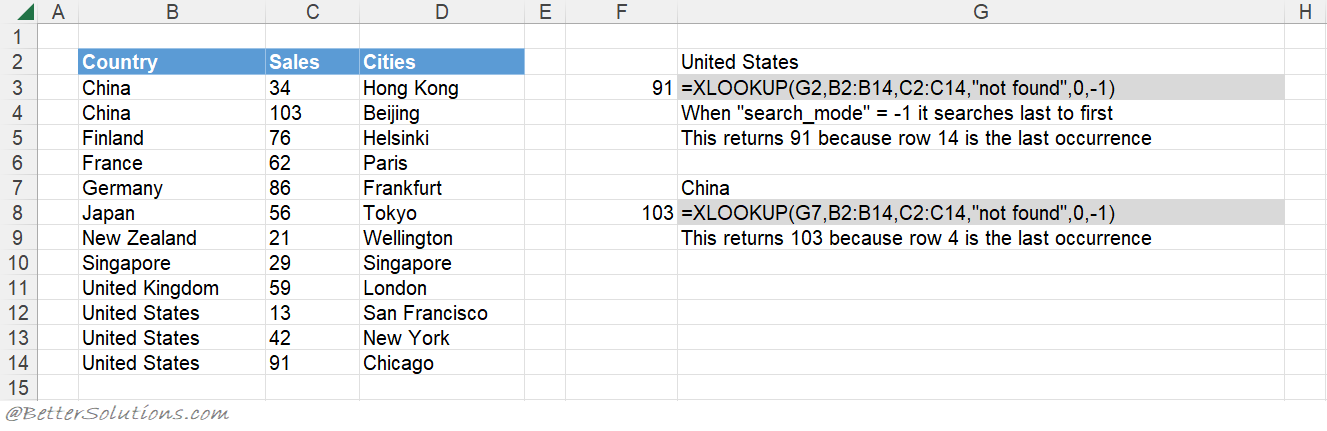### Return the Exact Match (last occurrence, not sorted)

The last occurrence can be achieved when the "lookup_value" column is not sorted.
When the "match_mode" argument is 0 an exact match is found. This is the default.
When the "search_mode" argument is -1 the last occurrence is found.
The table does not have to be sorted to return the last occurrence.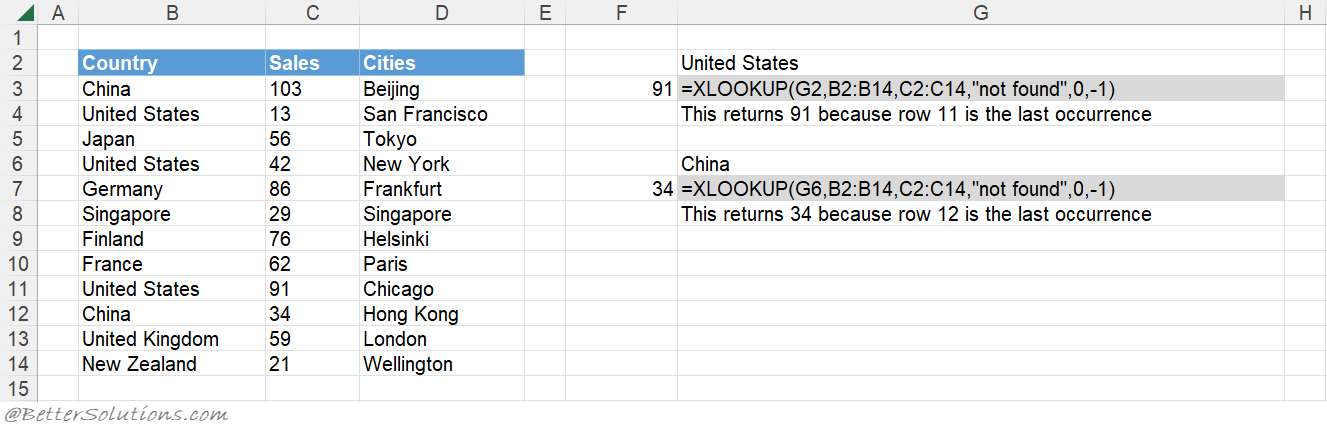### Return the Exact Match (with wildcard *)

An asterisk (*) matches any sequence of text characters (not numbers).
When the "match_mode" argument is 2 wildcard characters can be used.
You can have an asterisk as the first character.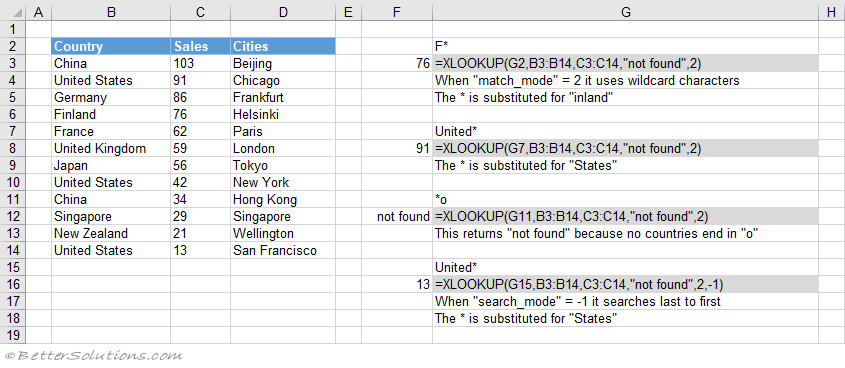### Return the Exact Match (with wildcard ?)

A question mark (?) matches any single text character (not numbers).
When the "match_mode" argument is 2 wildcard characters can be used.
You can have a question mark as the first character.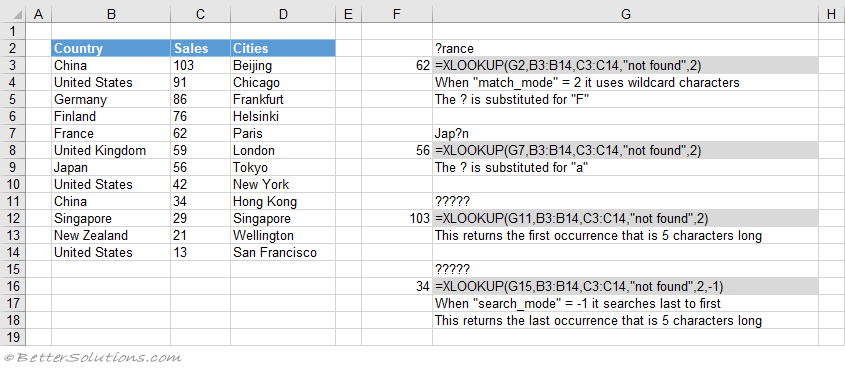### Return the Exact Match (multiple criteria)

When you want to return a value in the same row after finding matching values in 2 (or more) columns.

### Return the Closest Match (next smallest)

The next smallest value can be achieved when the "lookup_value" column is sorted into Ascending Order.
When the "match_mode" argument is -1 the next smallest value is found.
The table has been sorted by Sales.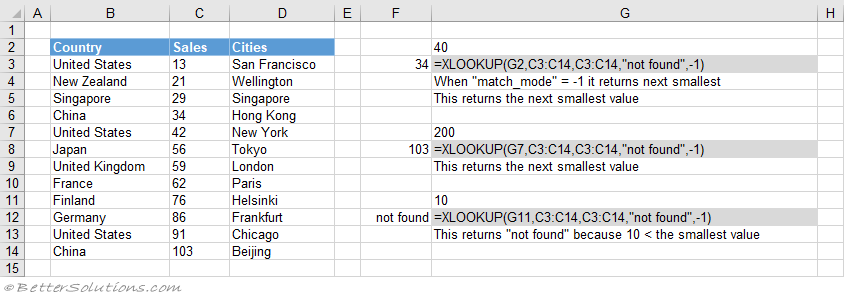### Return the Closest Match (next largest)

The next largest value can be achieved when the "lookup_value" column is sorted into Descending Order.
When the "match_mode" argument is 1 the next largest value is found.
The table has been sorted by Sales.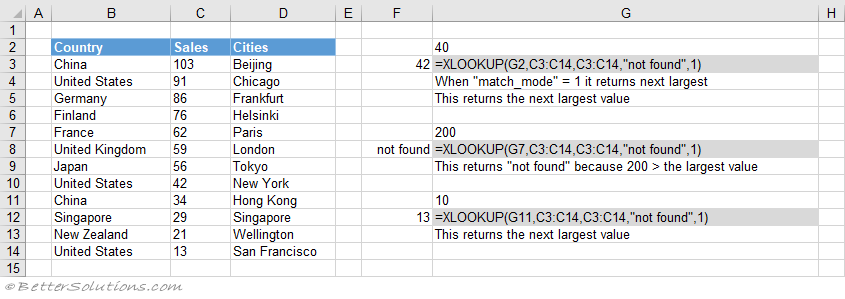### Combining with MATCH

A two-way lookup can be achieved using an XLOOKUP and MATCH combination.
A two-way lookup is when you return the intersection of a matching column with a matching row.